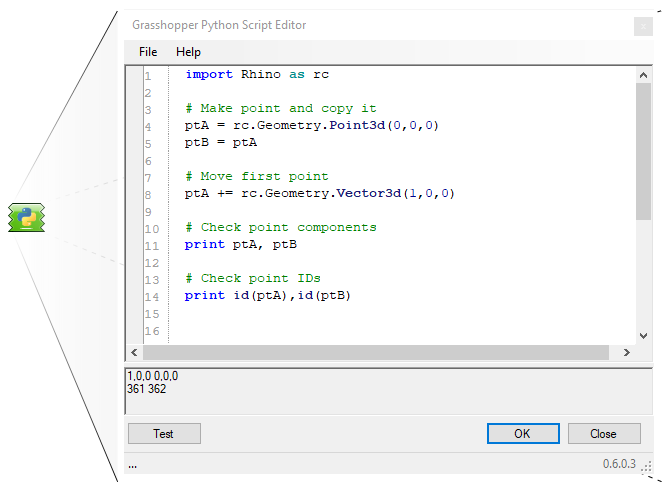# Box does it require duplicate method?

Hi,

For mesh, breps, curves classes, I duplicate objects by writing
`Mesh aCopy= a.Duplicate();`
For structs such as lines, points, it is enough to write
`Point3d aCopy = a;`

For Box objects is it also enough to write Box aCopy = a;
or it is must be somehow duplicated as well?

Passing a box to this constructor should do it:

https://developer.rhino3d.com/api/RhinoCommon/html/M_Rhino_Geometry_Box__ctor_1.htm

Thanks @AndersDeleuran

Is there a similar approach for Rhino 6 SDK?

Ah yes, no you’re right. I remember coming across this issue as well. My bad.

1 Like

Hi, I wrote some test codes for Point3d for both C# and Python and the results are interesting.
I want somebody more expert than me to confirm (and explain) this.

In C#,

``````var X=Transform.Translation(1,0,0);
var p=new Point3d(0,0,0);
var q=p;
p.Transform(X);
Print(p.ToString());
Print(q.ToString());
``````

This gives (1,0,0) and (0,0,0), whilst, in Python,

``````X=rg.Transform.Translation(1,0,0)
p=rg.Point3d(0,0,0)
q=p
p.Transform(X)
print p
print q
``````

this gives both (1,0,0).

For c# it makes sense because point3d is a Struct. for python there is something else Anders knows what

Structs don’t duplicate by assignment in IronPython like they do in C#. You need to explicitly duplicate them using e.g. the Python `copy` module, or the copy constructor if the struct has one (i.e. like `Box` has in V7):

https://developer.rhino3d.com/api/RhinoCommon/html/M_Rhino_Geometry_Point3d__ctor.htm

I try to use the explicit RhinoCommon struct option when possible. In the case of translating a point by a vector though I usually use the `+=` operator to add the two, which overwrites the value of the original, which negates the assignment copy issue in this case:Being explicit is probably always advisble though:2 Likes

Thank you! this is extremely helpful!

1 Like